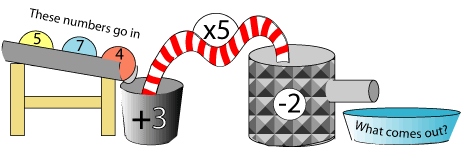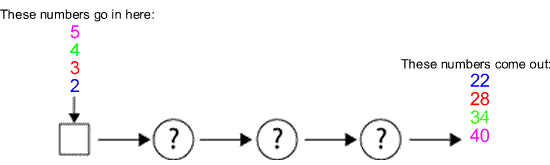#### You may also like### Exploring Wild & Wonderful Number Patterns

EWWNP means Exploring Wild and Wonderful Number Patterns Created by Yourself! Investigate what happens if we create number patterns using some simple rules.### Sending Cards

This challenge asks you to investigate the total number of cards that would be sent if four children send one to all three others. How many would be sent if there were five children? Six?### Dice and Spinner Numbers

If you had any number of ordinary dice, what are the possible ways of making their totals 6? What would the product of the dice be each time?

# Function Machines

## Function MachinesThis one is a bit more of a puzzle.Can you work out what happens in the three circles?

### Why do this problem?

This problem gives a good deal of practice in addition, subtraction, multiplication and division in an interesting and unusual format. The second part of the problem involves much reasoning about numbers.

### Key questions

Would it be a good idea to start with $5$?
What do you do next?
What comes out?
How could you get from $2$ to $22$ ? Will those same operations turn $3$ into $28$?
Would it be a good idea to multiply each number by $2$, then $3$, then $4$, etc until find a pattern?
What do you notice about the numbers going in? What do you notice about the numbers coming out?

### Possible extension

Learners could create their own function machines for friends to complete. Asking them to make examples like the second part of the problem will involve a great deal of mental calculation and reasoning about numbers.

### Possible support

Suggest trying just the first part of the problem which is relatively easy. They could then go on to making their own 'function machine' [as in the second part of the problem] with two, or even just one, circle.